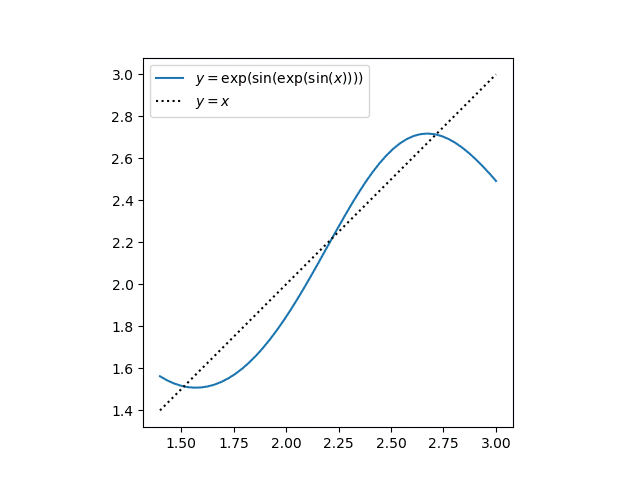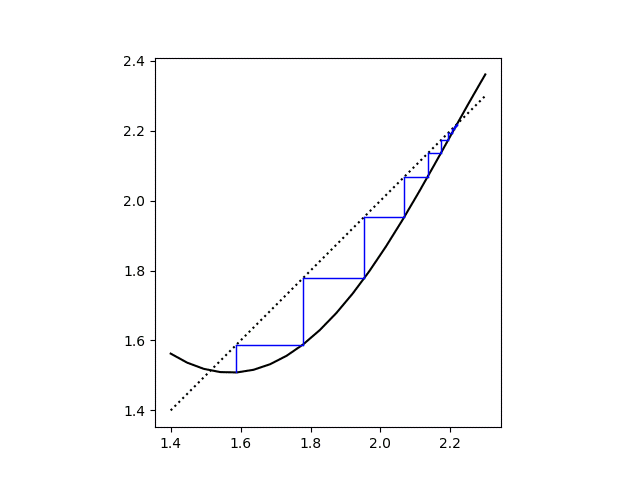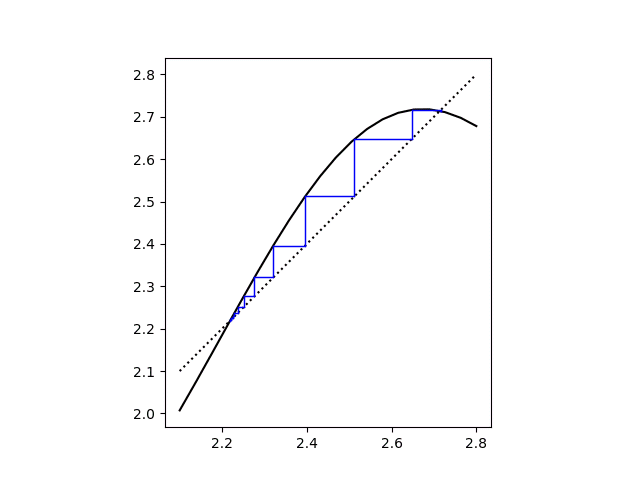# Square waves and cobwebs

This is a follow-up to yesterday’s post. In that post we looked at iterates of the function

f(x) = exp( sin(x) )

and noticed that even iterations of f converged to a square wave. Odd iterates also converge to a square wave, but a different one. The limit of odd iterations is the limit of even iterations turned upside down.

Jan Van lint correctly pointed out in the comments

If you plot y=f(f(x)) and y=x, you can see that there are two stable fixed points, with one unstable fixed point in between.

Here’s the plot he describes.You can see that there are fixed points between 1.5 and 2.0, between 2.0 and 2.5, and between 2.5 and 3. The following Python code will find these fixed points for us.

```    from numpy import exp, sin
from scipy.optimize import brentq

def f(x): return exp(sin(x))
def g(x): return f(f(x)) - x

brackets = [(1.5, 2,0), (2.0, 2.5), (2.5, 3)]
roots = [brentq(g, *b) for b in brackets]
```

This shows that the fixed points of g are

```    1.514019042996987
2.219107148913746
2.713905124458644
```

If we apply f to each of these fixed points, we get the same numbers again, but in the opposite order. This is why the odd iterates and even iterates are upside-down from each other.

Next we’ll show a couple cobweb plots to visualize the convergence of the iterates. We’ll also show that the middle fixed point is unstable by looking at two iterations, one starting slightly below it and the other starting slightly above it.

The first starts at 2.2191 and progressed down toward the lower fixed point.The second starts at 2.2192 and progresses up toward the upper fixed point.